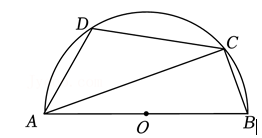$\text{A.}$ $100^{\circ}$ $\text{B.}$ $110^{\circ}$ $\text{C.}$ $120^{\circ}$ $\text{D.}$ $130^{\circ}$#### 解析：

①点击 首页查看更多试卷和试题 , 点击查看 本题所在试卷